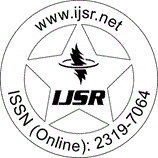International Journal of Science and Research (IJSR)
Call for Papers | Fully Refereed | Open Access | Double Blind Peer Reviewed

Research Paper | Computer Science & Engineering | Iraq | Volume 9 Issue 4, April 2020

# Modeling and Analysis of DC-DC Buck Converter for Mobile Applications

Mustafa M. Salih | Ahmed S. Al-Araji  | Hassan A. Jeiad 

Abstract: this paper presents the modeling of the DC-DC buck converter system based on the mathematical model and analysis the dc-dc buck converter system for the mobile device powered by fixed battery DC supply. The aim of the modeling is the mathematical representation of a power electronic circuit for a synchronous buck converter that controlled by fixed switching frequency in pulse width modulation. The state-space representation is used for modeling because of the population, and it is essential to define the state variable of a circuit, the state variable is the element storage of energy such as inductor and capacitor. This modeling included the parasitic resistances in the mathematical representation of power circuit elements. In this work, it analyzed and studied the stability and the dynamic behavior response of the buck converter output voltage model by using MATLAB/m. files simulation based on Bode plot frequency response and Root Locus method. This simulation illustrated the effective performance of the buck converter when switching frequency-changing, load resistance variation, and parasitic resistance changing for main inductor and capacitor through theoretical verification, graphical performance and MATLAB simulation.

Keywords: Buck Converter Model, State-Space, Parasitic Resistance, MATLAB Simulation

Edition: Volume 9 Issue 4, April 2020,

Pages: 1088 - 1093